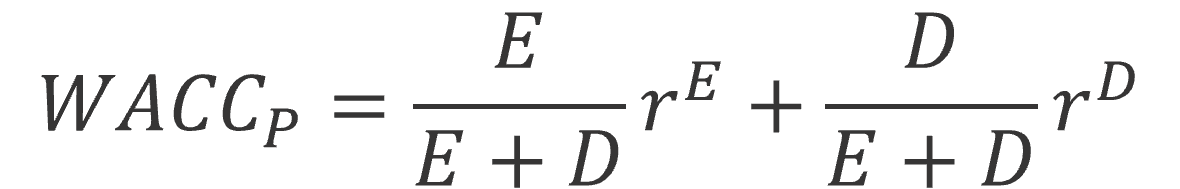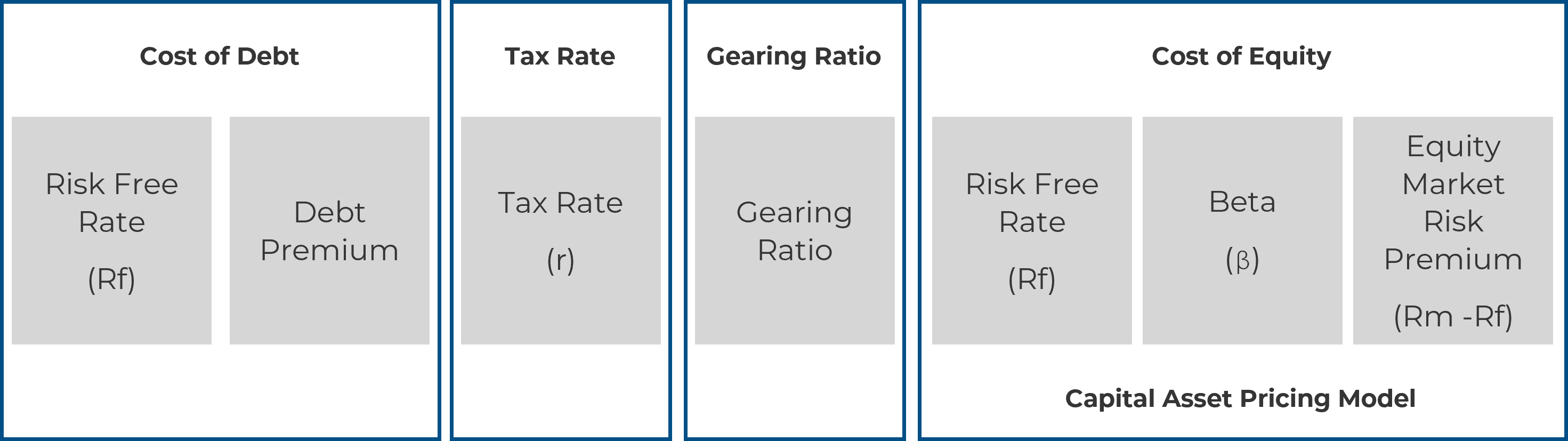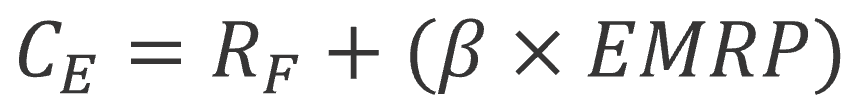Economic Evaluation of Projects

By Antonio J. Benavides and Francisco M. Benavides

Determining the Discount Rate to Use in Calculating the Present Value of Future Cash Flows

1. Cost of debt: The interest rate debt providers would charge the company for providing debt to such an investment.
2. Cost of equity: The rate of return equity investors would expect on an investment of this nature.
3. The gearing ratio: The relative proportions of debt and equity used to finance the investment.The parameters used in the calculations must be estimated or inferred from observable data.  The calculated WACC is thus an estimation based on assumptions and judgments and reference data. The following are the components of WACC formula:1. Cost of Debt

The cost of debt reflects the financing cost. The cost of debt is the sum of (1) the risk-free rate (Rf) and (2) the margin (premium) that lenders require above the risk-free rate, considering the risk & timeframe of the investment. This is the pre-tax cost of debt.

1.1. Determining the Risk-Free Rate

1.1.1. Risk-free means devoid of the following risks:

• Interest Rate Risk: changes in market interest rates.
• Inflation Risk: changes in inflation expectations.
• Default Risk: default on the repayment of the principal.
• Liquidity Risk: inability to liquidate the investment timely.
• Reinvestment Risk: i.e., inability to reinvest investment returns into new investments with similar return characteristics.

1.1.2. The risk-free rate can be determined by the yield of the Treasury bond matching your time horizon for the investment.

The additional return expected by lenders in the project to compensate for the above-described risks:

1.2.1. For projects undertaken by firms with a debt rating the corresponding spread can be directly determined.

1.2.2. If no debt rating is available, a synthetic rating and corresponding spread can be determined from the interest coverage ratio. This is because a firm’s debt premium will tend to increase with higher levels of gearing.

1.2.3. In both cases above, an adjustment should be made to the premium based on the relative risk of the project as compared to the company’s overall risk.

1.2.4. More debt equals more financial risk since the firm requires more cash flows to meet higher interest payments.

Adding the debt premium to the appropriate risk-free rate yields the pre-tax cost of borrowing for the firm to finance the new investment. Once calculated, the cost of debt should be adjusted by the tax shield to reflect how the interest payments on debt reduce the taxable profit of the investment, and hence reduces the tax liability and the effective post-tax cost of serving the debt.

2. Cost of Equity

The cost of equity is the return required by shareholders or individual investors on their investment as a form of compensation for the risk they bear by making such an investment.  The standard framework for calculating the cost of equity is the Capital Asset Pricing Model (CAPM).

The CAPM assumes an equity investor requires the investment to yield:

• At least the return available on risk-free investments PLUS.
• A premium for the risk involved in making an equity investment.

Total risk comprises two components:

2.1. Specific (diversifiable or idiosyncratic) risk—the risk specific to a particular firm /investment that can be diversified away by investors and thus is not priced into investor’s required rates of return.

2.2. Systematic (or undiversifiable) risk—how the value of an asset covaries with the economy as a whole and cannot be diversified away by investors, as it tends to have some impact on all firms / investments.

CAPM assumes the required return need reflect only the systematic risk derived by reference to the volatility of the returns on the particular firm / investment relative to those of the market as a whole. The CAPM formula follows:where:

Cis the cost of equity: a return required by equity investors (expressed as percentage).

RF is the risk free rate.

ß is the beta coefficient: a measure of the extent to which returns on the regulated firm’s shares co-vary with the returns of the market as a whole.

EMRP is the Equity Market Risk Premium: the additional return required for investing in the equity market compared to investing in risk-free assets.

In particular, an investment’s beta can best be determined by looking at the betas of comparable investments and with respect to its specific project risk characteristics.

Finally, if relevant, the country risk premium should also be weighed into the total risk premium:

• Reflects the inherent risks of investing in particular countries.
• Reflects differences in the level of financial, political, economic, and institutional stability of different countries.
• Close to zero for most developed and stable countries.

3. The Gearing Ratio

Please click on the link below for an article regarding determination of the optimal capital structure (most effective mix of debt and equity) for a major capital investment.

The Optimal Capital Structure for Financing an Industrial Project

At PEC Consulting Group we work with each of our customers to adapt a tailored approach to structure the best financing solution for new industrial projects, determined by the specific characteristics and objectives laid forth.